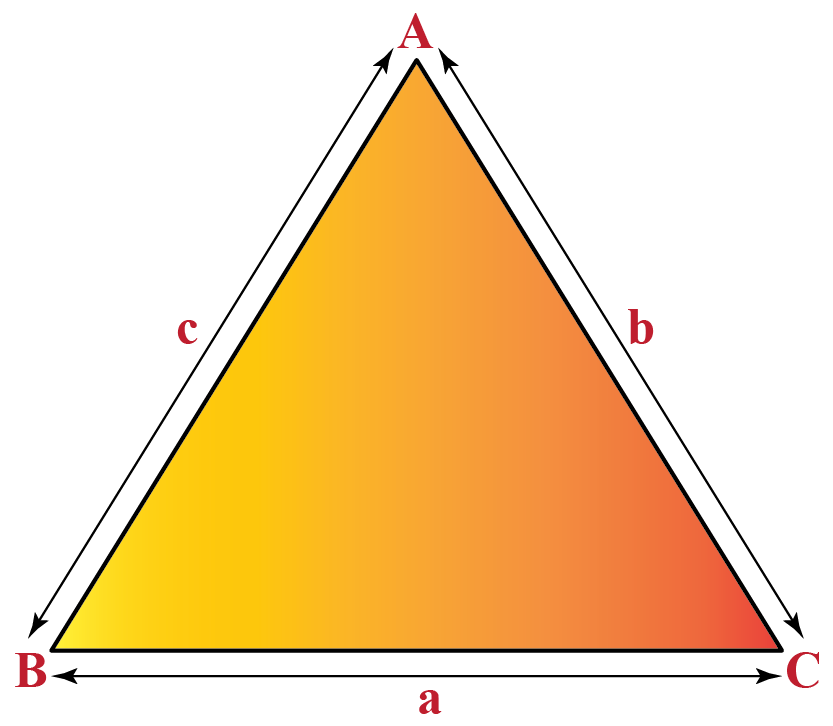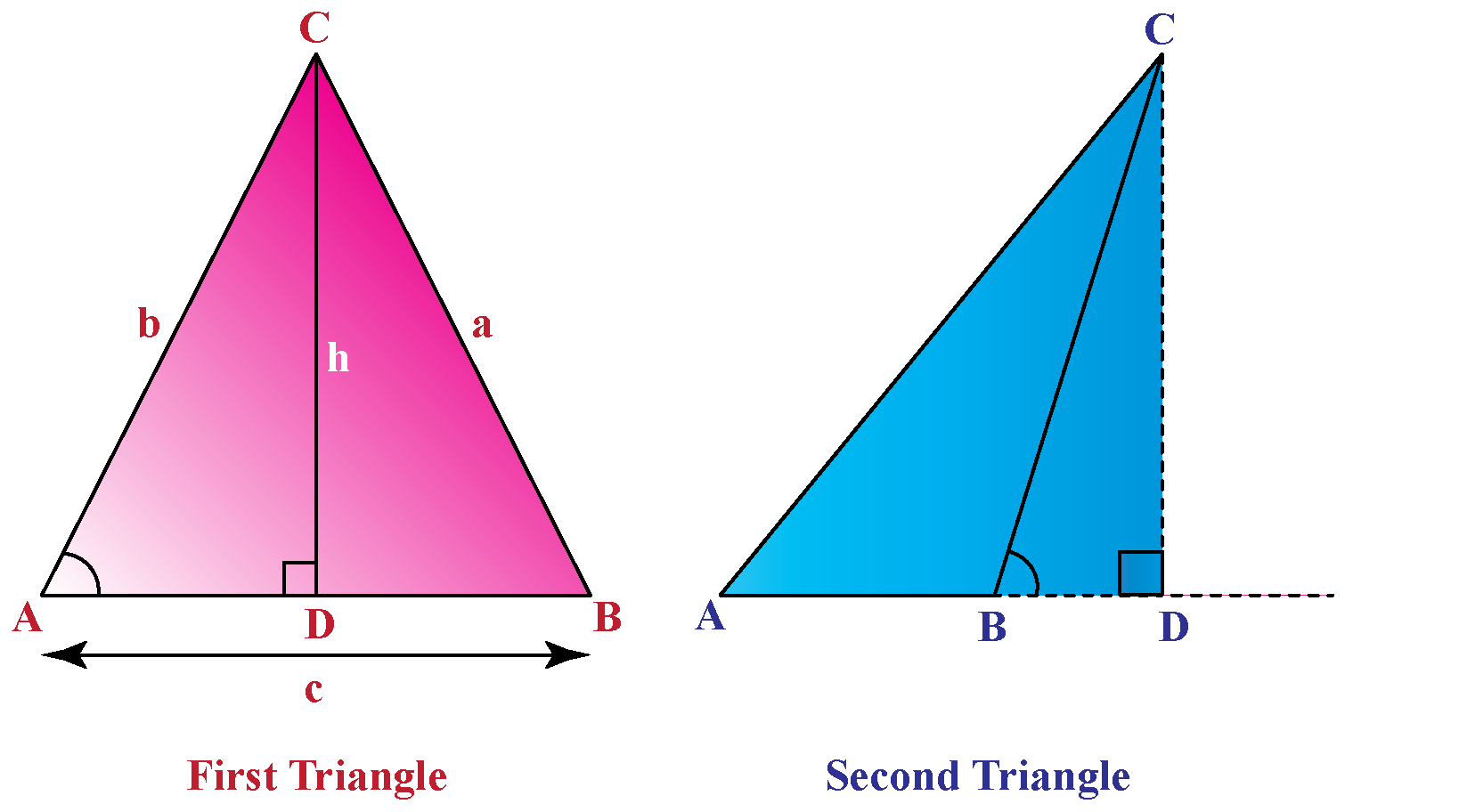# Sine Law

Sine Law

We have been always applying trigonometric ratios to right-angled triangles. Here we shall use the same trigonometric ratios for oblique triangles also.In this mini-lesson, we shall explore the topic of sine law, by finding answers to questions like what is law of sines, what are the applications of sine law, and proof of the sine law.

## Lesson Plan

 1 What Is Law of Sines? 2 Tips and Tricks 3 Think out of the Box! 4 Solved Examples on Law of Sines 5 Interactive Questions on Law of Sines

## What Is Law of Sines?

### Definition

The ratio of the side and the corresponding angle of a triangle is equal to the diameter of the circumcircle of the triangle.

### Formula

The formula for sine law is:

$\dfrac{a}{SinA} = \dfrac{b}{SinB} = \dfrac{c}{SinC}$• Here a, b, c are the lengths of the sides of the triangle.
• A, B, and C are the angle of the triangle.
• R is the radius of the circumcircle of the triangle.

## Proof of the Sine Law

To prove the sine law, we consider these two oblique triangles.In the first triangle, we have:

\begin{align}\dfrac{h}{b} &= SinA\\h&=bSinA\end{align}

In the second triangle, we have:

\begin{align}\dfrac{h}{a} &= SinB\\h&=aSinB\end{align}

Also $$Sin(180^\circ - B) = Sin B$$.

Equalizing the h values from the above expressions, we have:

\begin{align}aSinB&=bSinA\\\dfrac{a}{SinA}& = \dfrac{b}{SinB}\end{align}

Similarly, we can derive a relation for $$SinA$$ and $$SinC$$.

\begin{align}aSinC&=cSinA\\\dfrac{a}{SinA}& = \dfrac{b}{SinB}\end{align}

Combining the above two expressions, we have the following sine law.

\begin{align}\dfrac{a}{SinA}& = \dfrac{b}{SinB} = \dfrac{c}{SinC}\end{align}Tips and Tricks
1. Triangulation Technique is used to find the sides of a triangle when two angles and one side of a triangle is known. For this the sine law is helpful.
2. This sine law of trigonometry should not be confused with the sine law in physics.
3. Further deriving from this sine law we can also find the area of an oblique triangle. $\text{Area of a triangle}=\dfrac{1}{2}abSinC = \dfrac{1}{2}bcSinA = \dfrac{1}{2}caSinB$
4. Also sine law provides a relationship with the radius R of the circumcircle.  $\dfrac{a}{SinA} = \dfrac{b}{SinB} = \dfrac{c}{SinC} = 2R$

## What Are the Applications of Sine Law?

The sine law can be applied to calculate:

• The length of the side of a triangle
• The unknown angle of a triangle
• The area of the triangleThink Tank

COSINE LAW:  This proves a relationship between the sides and one angle of a triangle.  $c^2 = a^2 + b^2 - 2ab \cdot CosC$

TANGENT LAW: This has been derived from the sine law and it gives the relationship between the sides and angles of a triangle.  $\dfrac{a - b}{a + b} = \dfrac{Tan\frac{(A - B)}{2}}{Tan\frac{(A + B)}{2}}$

Q. Find the angles of a triangle if the sides are 12 units, 8.5 units, and 7.2 units respectively.

## Solved Examples

 Example 1

Given a = 20 units c = 25 units  and Angle C = $$42 ^\circ$$. Find the angle A of the triangle.

Solution

For the given data, we can use the following formula of sine law. $\dfrac{a}{SinA} = \dfrac{b}{SinB} = \dfrac{c}{SinC}$

\begin{align}\dfrac{20}{SinA} &= \dfrac{25}{Sin42^\circ} \\\dfrac{SinA}{20} &= \dfrac{Sin42^\circ }{25}\\ SinA&= \dfrac{Sin42^\circ}{25} \times 20\\ SinA&= \dfrac{Sin42^\circ}{25} \times 20\\SinA&= \dfrac{0.6691}{5} \times 4\\SinA &= 0.8363\\ A &= Sin^{-1}0.8363 \\A &=56.7^\circ \end{align}

 $$\therefore \angle A = 56.7^\circ$$
 Example 2

Two angles and an included side is . $$\angle A = 47^\circ$$ and $$\angle B = 78^\circ$$ and c = 6.3 units. Find the value of a.

Solution

Given  $$\angle A = 47^\circ$$ and $$\angle B = 78^\circ$$

\begin{align} \angle A + \angle B + \angle C &= 180^\circ \\47^\circ + 78^\circ + \angle C&= 180^\circ \\ 125^\circ + \angle C &= 180^\circ \\ \angle C &= 180^\circ - 125^\circ \\ \angle C &= 55^\circ\end{align}

We shall apply the sine law to find the side of the triangle.
\begin{align}\dfrac{a}{SinA} &= \dfrac{c}{SinC} \\\dfrac{a}{Sin47^\circ} &= \dfrac{6.3 }{Sin 55^\circ}\\ a&= \dfrac{6.3}{Sin 55^\circ} \times Sin 47^\circ\\ a &= \dfrac{6.3}{0.8192} \times 0.7314 \\a &= 5.6 \end{align}

 $$\therefore a = 5.6 units$$

## Interactive Questions on Law of Sines

Here are a few activities for you to practice. Select/Type your answer and click the "Check Answer" button to see the result.

## Let's Summarize

The mini-lesson targeted the fascinating concept of sine law. The math journey around sine law starts with what a student already knows, and goes on to creatively crafting a fresh concept in the young minds. Done in a way that is not only relatable and easy to grasp, but will also stay with them forever. Here lies the magic with Cuemath.

## About Cuemath

At Cuemath, our team of math experts is dedicated to making learning fun for our favorite readers, the students!

Through an interactive and engaging learning-teaching-learning approach, the teachers explore all angles of a topic.

Be it worksheets, online classes, doubt sessions, or any other form of relation, it’s the logical thinking and smart learning approach that we, at Cuemath, believe in.

## FAQs on Sine Law

### 1. What is meant by law of sines?

Law of sines gives a relationship between the sides and angles of a triangle.  $\dfrac{a}{SinA} = \dfrac{b}{SinB} = \dfrac{c}{SinC}$

### 2. When can we use sine law?

We can use the sine law to find:

• Side of a triangle
• The angle of a triangle
• Area of a triangle

### 3. What is the sine rule?

The sine rule gives the ratio of the sides and angles of a triangle.
$\dfrac{a}{SinA} = \dfrac{b}{SinB} = \dfrac{c}{SinC}$
Here a, b, c are the length of the sides of the triangle, and A, B, C are the angles of the triangle.

### 4. What are the different ways to represent sine rule formula?

Sine law can be represented in the following three ways.

1. $$\dfrac{a}{SinA} = \dfrac{b}{SinB} = \dfrac{c}{SinC}$$
2. $$\dfrac{SinA}{a} = \dfrac{SinB}{b} = \dfrac{SinC}{c}$$
3. $$\dfrac{a}{b} = \dfrac{SinA}{SinB}; \dfrac{a}{c} = \dfrac{SinA}{SinC};\dfrac{b}{c} = \dfrac{SinB}{SinC}$$

### 5. In which cases can we use the sine law?

The sine law can be used for three purposes.

1. To find the length of sides of a triangle
2. To find the angles of the triangle
3. To find the area of the triangle

### 6. Can sine law be used on a right triangle?

The sine law can also be used for a right triangle.

### 7. What are the possible criteria for law of sines?

The criteria to use the sine law is to have the following.

A pair of lengths of two sides of a triangle and an angle.

OR

A pair of angles of a triangle and the length of one side.

Download Trigonometry Worksheets
Trigonometry
Grade 10 | Answers Set 1
Trigonometry
Grade 9 | Questions Set 1
Trigonometry
Grade 9 | Answers Set 1
Trigonometry
Grade 10 | Questions Set 1
More Important Topics
Numbers
Algebra
Geometry
Measurement
Money
Data
Trigonometry
Calculus